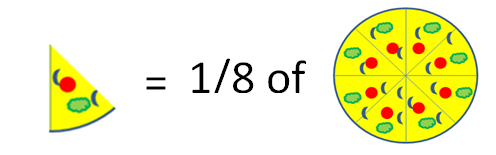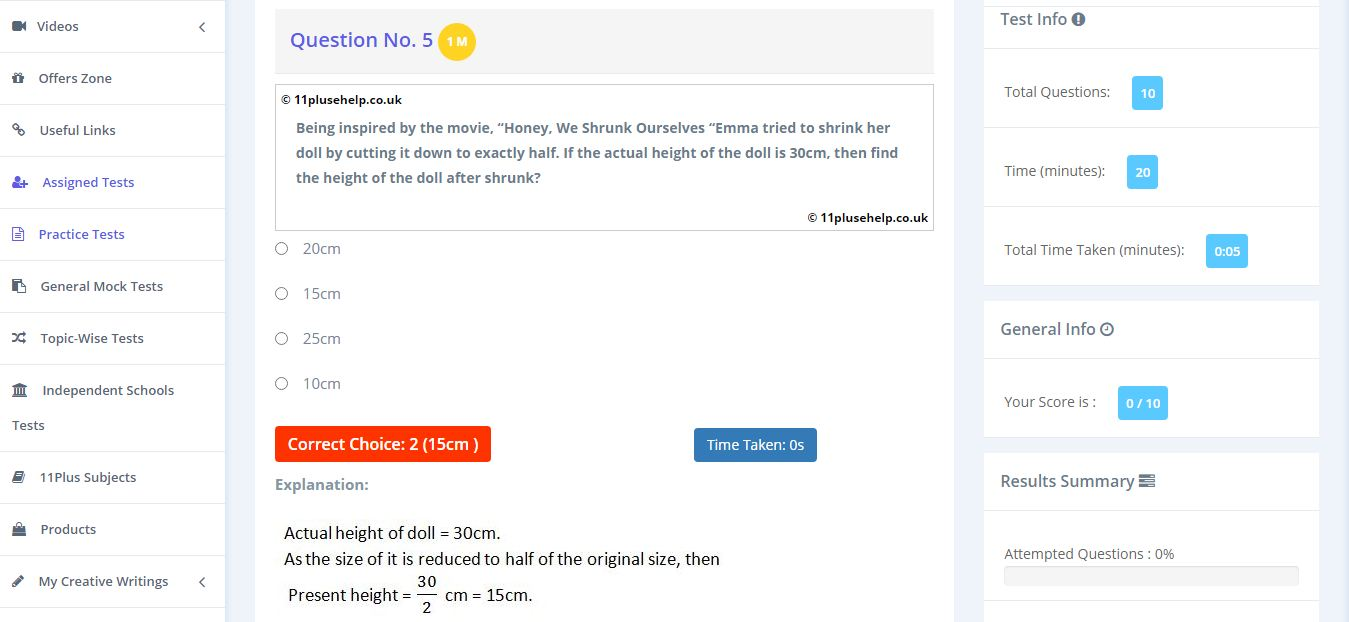Latest Results:

# 11 Plus Fractions

Fractions are considered as representing a part of a whole quantity. Fractions are usually represented in the form of a/b where a, b are 0. The terminologies of fractions are generally used while dividing a thing into smaller parts, like sharing a pizza among friends.The pizza shown in the above picture has been divided into eight pieces. When the pizza is considered a whole, each part is given by 1/8, which is a fraction.A fraction consists of 2 parts they are Numerator and Denominator.Types of fractions:

Generally, fractions can be divided into three types they are

• Proper Fractions
• Improper fractions
• Mixed Fractions

The fractions in which the denominator is greater than the numerator, then such fractions are considered as the Proper Fractions. Examples are ½, ¼, ¾, etc.

The fractions in which the denominator is greater than the numerator, then such fractions are considered as the Improper Fractions. Examples are 11/4, 5/2, 10/7 etc.

The other form of representing an improper fraction is in terms of Mixed Fractions. It is a combination of a whole number and a proper fraction. Examples are 3 ½, 5 ¼, 7 ¾, etc.

Some important facts about fractions are

• The fractions with the same denominator are said to be Like Fractions else, they are said as, Unlike Fractions.
• A fraction in which numerator and denominator are equal, then its value will be equal to One. Eg 2/2 = 1.
• A fraction in which the numerator = 0, then the value of the fraction will be Zero. Eg 0/100 = 0.
• A fraction in which the denominator = 0, then the fraction is said to be undefined.

Example: Jason brings a cake and cuts it into 6 equal parts and distributes among his friend, what fraction does each friend get?

Sol: No. of pieces = 6, then each one will be getting 1/6 part of the cake.

Arithmetic operations on fractions

To perform addition or subtraction, we need to check if the fractions have the same denominator or not. We will know about them in detail through some interesting and easy examples.

Consider two fractions, a/b and c/b, and then their sum will be (a + c)/b, similarly in subtraction also, it will be (a-c)/b. Here denominators of both the fractions are equal.

Now Consider two fractions, a/b and c/d. Then, to find their sum or difference, we must make their denominators equal. For this, we must find the LCM of the denominators, and based on it, and we must make the fractions like or we follow another method, in which the fractions are represented as (ad/bd), (bc/bd) and perform the addition or subtraction.

Sol: First, convert them into like fractions. 1/2 can be written as (1x3) / (2x3) = 3/6. Similarly, 2/3 can be written as 2/6. Now we can add both the fractions,

3/6 + 2/6 = 5/6

For multiplication, it is very easy, multiply the numerators and denominators separately. For division, reciprocate the 2nd fraction and multiply.

Multiplication of a / b & c / d = (a x b) / (c x d)

Division of a / b & c / d = (a x d) / (b x c)

Example: Multiply 1/4 and 2/3?

Sol: It is very easy to multiply numerators and denominators separately.

1/4 x 2/3 = (1 x 2) / (4 x 3) = 2 / 12.

11 plus fractions topic is very important as it will be used to calculate percentages and decimals and many other 11 pus maths topics. You can access 11 plus maths practice tests on different topics here:

https://www.11plusehelp.co.uk/11-plus-maths-practice-tests

Try out these examples without sneak peeking at the answers:

Example:1Example:211PluseHelp.co.uk is a one-stop-shop for 11 Plus exam preparation, including 11 Plus Independent schools preparation.

There are many 11 Plus practice papers for entrance exams, Independent school papers and more for the 11 test with us. Ranging from 10 to 25 minutes, our multiple-choice and fill-up question types are ideal for Maths revision.

At 11plusehelp.co.uk/, we possess the following resources:

Maths- 11 Plus Maths Worksheets, 11 Plus Maths Papers free download, 11 Plus Maths topics pdfs.

English- 11 Plus English papers with answers, 11 Plus comprehension with answers, 11 English comprehensions multiple-choice, English reasoning test.

Verbal and Non-Verbal Reasoning- 11 Plus Verbal Reasoning worksheets free, Verbal and Non-Verbal Reasoning tests, easy Non-Verbal Reasoning Worksheets

Practice Tests- Eleven plus sample papers, free 11 plus papers with answers, 11 plus mock test papers pdf, 11 exam question and answers, UK 11 plus exam questions, 11 online tests in Maths and English with 11 Plus answers.

To access Virtual Mock Exams, please visit:  https://11plus-mockexams.co.uk/

You can access 11 Plus FREE Papers by visiting the below link:
https://www.11plusehelp.co.uk/11-plus-free-online-papers

You can access 11 Plus FREE Sample Papers by visiting the below link:
https://www.11plusehelp.co.uk/11-plus-sample-papers

11 Plus complete solution features:

https://11plusehelp.co.uk/blog/2017/05/01/11-plus-complete-solution/

Practice and Perseverance Over Genius and Talent

Thanks,

11Plusehelp.co.uk